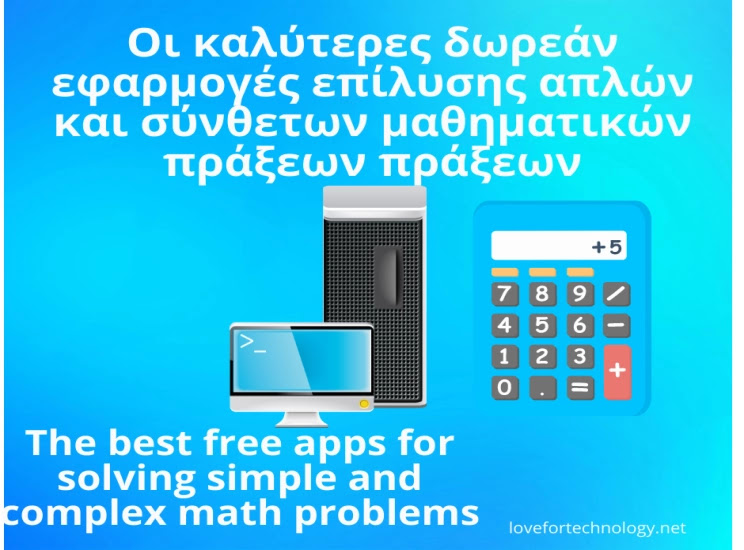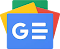## Wednesday April 26 2023As we all know, in all versions of windows, the classic computer (calculator) that helps us perform mathematical operations has remained almost the same for many years without much emphasis being given to its development.

Therefore for those who want to do complex mathematical operations such a thing is impossible. If you search, you will definitely find several third-party applications that can fill this gap, and in fact, several of them are available completely free.

Today, we present the 21 best math problem solving apps, hoping to cover everyone from the most ignorant to the very advanced.

CONTENTS

### Secyon calculator

1.

All-in-one scientific calculator for every student or teacher, researcher or developer - everyone doing physics or chemistry / science or engineering using formulas and tables of constants.

It's easy to use and has everything you need to calculate or investigate a function - graph, roots, min/max, integrals, derivatives, fit data, etc.

The Sicyon calculator combines an intuitive interface and an object-oriented approach with a fast learning curve while remaining at a high level of performance.

Sicyon Calculator is free software licensed under Creative Commons by-nv-nd-3.0. & part

### calculate

2.

Calculator for Windows, multipurpose. Simple to use, but provides power and flexibility usually reserved for complex math packages, as well as useful tools for everyday needs (such as currency conversion and percentage calculation).

Features include a large library of customizable functions, unit calculations and conversions, physical constants, symbolic calculations (including integrals and equations), arbitrary precision, uncertainty propagation, interval arithmetic, plotting, and a user-friendly interface (GTK, Qt, and CLI) .

### Speedcrunch

3.

High precision scientific computer. It is free and open source software, licensed under the GPL.

SpeedCrunch displays the results as you type. It includes more than 80 built-in math functions, however, you can also define your own.

Variables allow you to store your results, perform calculations with up to 50 digits of precision, using complex numbers, arithmetic bases, unit conversions, and more.

### eCalc Scientific

4.

Easy-to-use scientific calculator that supports many advanced features such as unit conversion, equation solving and complex math.

eCalc is offered as both an online calculator and a downloadable calculator. Works with either algebraic input (default mode) or RPN input.

The algebraic input mode is commonly referred to as "infix notation" and is widely used by most handheld computers.

Expressions entered in algebraic input mode are done in a way that closely resembles the natural form of the expression, and the order of operations is determined by operator precedence and parentheses.

### Calculator²

5.

Scientific advanced calculator with a comprehensive set of mathematical functions including, trigonometric, hyperbolic, logarithmic, exponential. It has over 125 mathematical and physical constants.

A calculator with a variety of functions for performing financial calculations, such as determining the monthly payments for a loan, even can be set up to mark up, and discount, taxes and interest.

Ideal for students and professionals, however, the currency converter is unlocked by an in-app purchase, which also removes ads.

### HEXELON MAX

6.

HEXelon MAX is a complete scientific calculator with many possibilities that, among other things, includes exchange rates and currency conversions, as well as various other tools. Numerical systems: binary, octal, decimal, hexadecimal. Shows routines, variables, constants.

### Calc Pro HD

7.

Make your own calculator! Choose only the calculators and features you need to customize with Calc Pro HD! Choose up to 8 calcs to create the perfect calculator!

The best portable calculator is now available for Windows 10. The only calculator in the Windows store that can truly replace the classic pocket calculators.

Calc Pro HD offers more power, more features, more accuracy and is by far the top choice for both professional and home use needs.

Use the power of Cortana to perform your calculations. However, I must note that in its free version it includes only two calculators, the classic and the scientific.

### HYPER CALC

8.

It is an impressive scientific calculator that will cover even the most demanding since it has an advanced function and can process and solve many mathematical problems arising from operations between powers, roots, logarithms, fractions, hyperbolic functions and many others.

### Math Editor

9.

Math Editor is an equation editor with the help of which you can create, save and edit equations through a simple and friendly environment.

The new version of Math Editor includes more features than previous versions with better support for creating equation templates.

To be able to download the application you must register on the website of its creator.

### RedCrab The Calculator

10.

Scientific calculator with a full editor.

Mathematical commands are not standardized on a standard command line, but can be entered in a window similar to a piece of paper.

The computer displays the result either below or next to it. It is easier to operate than conventional calculators.

### Calculations

11.

Free scientific calculator with functions, which can be used especially by high school students or college students.

It offers you a wide range of tools to make your calculations easier and faster.

Its features include the ability to evaluate complex expressions with parentheses, variables, constants, functions, calculations in four systems, binary, octal, decimal, hexadecimal, transcendental functions, graphs of functions, calculations with polynomials and fractions, and more.

### Symbolab - Math solver

12.

Gone are the days when students searched for solutions to difficult problems in lysarias.

Nowadays, thanks to the development of technology, we can look for the solutions to difficult mathematical problems through specially designed programs made for computers.

In our case, things are not exactly like that since the solution to student equations and other problems comes through the internet.

More specifically, the symbolab website is a first class opportunity for the older ones to remember and the younger ones to practice math.

What we should do is simply type or click the relevant mathematical symbols depending on the operation we want to do and of course find the solution.

One of the most interesting features of the application is that it allows us to enter characters or symbols that do not exist in the usual keyboards of a simple computer and perform any action we wish.

### Euler Math Toolbox

13.

Euler Math Toolbox (EMT), a powerful math program that combines arithmetic and symbolic tools (using the Maxima computer algebra system) into a free symbolic algebra package, with which one can do analytical linear algebra calculations.

So if you are a student, teacher, professor, or just love math and are looking for a powerful, easy-to-use yet free Matlab replacement, then this program is exactly what you need.

### SMath Studio

14.

Mathematics program in a natural environment, in the sense that the arithmetic operations we do are done on a ΄΄paper΄΄ and includes many possibilities.

It has the ability to analyze and solve vectors, complex numbers, inverse trigonometric functions, logarithms, with the ability to display 2D or 3D graphs, unlimited size of worksheets, fractions and more, also includes versions for Windows, and Linux.

In addition to its online form, the program is also available as a stand-alone program. It has a multilingual support environment for 35 languages, including Greek.

15.

### caligator

It is a simple, opensource, crossplatform calculator for all your calculation needs that allows you to do unit calculations and conversions, with features like, weight conversion, length conversion, temperature conversion, currency conversion.

Very simple simple to use since it works exactly the way you think.

### Alternate Calculator

16.

This is a simple calculator that can also be used for currency exchange.

It already includes a set of predefined currencies and exchange rates, which can also be modified individually.

An internal processor is included which can be used to rebind and save already performed calculation steps. The calculator can also be used to work between different number systems (decimal, hexadecimal, binary, customer-defined).

The program is customizable in window size and appearance.

The program needs the .NET-framework 2.0 or higher (which is already included in Windows since Windows Vista).

### Naturplay Calculator

17.

Powerful, stylish general purpose calculator for Windows 11. It supports step by step calculation until the answer is reached.

This is a calculator without the switch between simple and scientific mode since it includes simple and extended functions in the same menu.

In addition, tables, statistics and complex functions can be applied with a user-friendly experience. As you type, answers can be extracted instantly.

In addition to enlarging the calculation result table, you can also save the calculation results as images for use or document visualization.

### fx-Calc

18.

fx-Calc is actually one of the most advanced calculators for computers running Windows. Its great advantage is the simple and intuitive handling of complex mathematical tasks.

Starting with the familiar calculator window, it allows the calculation of self-defined functions and sums.

For example, it only takes a few clicks to run the Leibnitz iteration to calculate Pi. fx-Calc allows you to define, analyze, visualize and calculate your scientific math operations.

In addition to calculating and visualizing results you can analyze and solve scientific functions and perform linear regression and direct calculation of functions with up to 5 variables, you can solve equations and many other complex mathematical operations.

### GeoGebra

19.

Free dynamic math software for all levels of education that integrates geometry, algebra, spreadsheets, graphing, statistics, and calculus into one easy-to-use package.

Interactive learning, teaching and assessment projects created with GeoGebra can be shared and used by anyone. It is the world's favorite dynamical mathematics program.

As educational software it has numerous awards and supports STEM educational innovation in teaching and learning.

### Maxima

20.

Maxima is a descendant of Macsyma, the legendary computer algebra system developed in the late 1960s at the Massachusetts Institute of Technology.

It is the only system based on this effort that is still publicly available and with an active user community, thanks to its open source nature.

Macsyma was revolutionary in its time, and many later systems, such as Maple and Mathematica, were inspired by it.

Maxima is a system for handling symbolic and numerical expressions, including differentiation, integration, Taylor series, Laplace transforms, ordinary differential equations, systems of linear equations, polynomials, sets, lists, vectors, matrices, and tensors.

Returns high-precision numerical results using exact fractions, arbitrary-precision integers, and variable-precision floating-point numbers, and can plot functions and data in two and three dimensions.

### Simplexity

21.

Free software utility designed specifically to help you perform various mathematical operations. Whether you're a math teacher, student, or engineer, you'll find that this app is more than just a simple scientific calculator.

With comprehensive and rich graphical interface, at first glance, the application interface may confuse you because it contains many buttons, mathematical expressions, symbols and tabs, especially if you are a novice user. But once you get used to its layout, you can easily perform complex operations with just a few clicks.

It supports many dedicated parameters that allow you to perform various mathematical operations such as subtraction, addition, division, multiplication, as well as trigonometric, parametric or polar functions.

You can also calculate integrals, derivatives, and multiple equations based on well-known abstract mathematical theories found in science and math textbooks.

You can also edit equations according to your needs, but keep in mind that to use Greek characters, you have to double-click on each symbol. Overall, Simplexety is a sophisticated software application unlike what its name might suggest.

Know of any others you'd like us to add to our collection?Follow us on Google News

TAGS: Collections